ChemTalk

# Isotope Abundance and Average Atomic Mass

## Core Concepts

When looking at the periodic table, each element has a value displayed for the atomic mass. If you look closely, it is clear that these values are almost never whole numbers. This is due to isotope abundance. In this tutorial, we will learn what isotope abundance is and how to use it to calculate the atomic weight of an element.

## Vocabulary

• Isotope: when an element has a different form in which it contains the same number of protons, but differs in the number of neutrons.
• Proton: Positively charged subatomic particle located in the nucleus of an atom.
• Neutron: Neutrally charged subatomic particle located in the nucleus of an atom.

## What is an isotope?

Isotopes are very similar versions of the same element, only having one difference: the number of neutrons. Though these two versions of the same element differ in the number of neutrons, it is important to note that they do not differ in the number of protons and electrons. In some instances, isotopes can have different reactivity, but in most cases, the defining difference is the number of neutrons.

A common example of an isotope having reactivity that differs from what the element is known for is carbon. Carbon is known to be a very stable element, often being involved in predictable reactions. One isotope of carbon, carbon-14, defies the normal reactivity of the stable element. Carbon-14 is a naturally occurring carbon isotope that radioactively decays. Read more about carbon here.

## How does isotope abundance impact atomic weight?

Atomic mass depends on the composition of protons and neutrons in an element, with each weighing 1 atomic mass unit (amu). Electrons are an important part of elements as well, but they have such a small mass that they are considered negligible when calculating atomic mass. As both protons and neutrons make up an atom’s mass, when an element differs in its number of neutrons, it is impactful on the mass.

Though they sound like synonyms, atomic mass and atomic weight are different. Isotopes impact the value of both. Atomic mass is defined as the mass of an individual atom of an element. This is solely the calculation of the weight of protons and neutrons in amu. Atomic weight on the other hand is the weighted average of all of the isotopes of an element that exist. This is where isotope abundance comes in. Though there may be many naturally occurring isotopes of an element, they do not exist in equal amounts. There are many isotopes that occur much more commonly than others, and therefore have a greater impact on the atomic weight. If given the atomic mass of the isotopes of an element as well as their relative abundances, we can follow simple steps to calculate the atomic weight.

## Using isotope abundance to calculate atomic weight

As stated previously, the number of isotopes and their percent abundance are all that are needed to calculate the atomic weight of an element. We can start by using magnesium as an example. Magnesium has three naturally occurring isotopes: 24Mg, 25Mg, and 26Mg. Each isotope has an abundance of 78.70%, 10.13%, and 11.17%, respectively. The atomic mass of each isotope is usually very close to each isotope value. In this example, the mass of each isotope is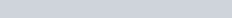, and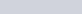respectively.

Now that we have all of the information about mass and abundance, we can calculate the atomic weight of magnesium. If you have trouble visualizing all of the values, you can organize them in a table to make your information more clear.

We start by multiplying each isotopes’ mass by its abundance. This can be done in two ways. First, we can directly multiply the mass by the percent: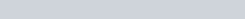On the other hand, we can change the percent to a decimal out of one and then multiply by the mass. This can be done by dividing the percent by 100.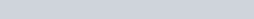With both of these methods, the next step is to repeat for the other isotopes and add the values together.

##### Method 1: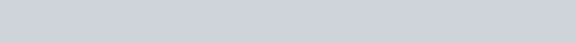##### Method 2: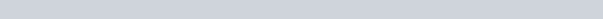If you follow method one, the final step is to divide the product by 100 to compensate for the percentages being whole numbers.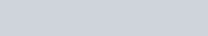Now that we have obtained the same value for both methods, the last step is to make sure your answer has the correct number of significant figures. In this case, the final value is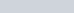.

## Other Examples

Now that you have seen a general example of how to calculate atomic mass from isotope abundances, we can understand other problems involving isotope abundance.

##### Calulating abundance form atomic mass and atomic weight

Lithium has two isotopes, 6Li and 7Li, with masses of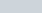and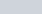respectively. If Lithium has an atomic weight of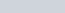, we can determine the abundance of each isotope. First, we define one. of the abundances as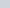. In this case, the abundance of 6Li will be. This means that the abundance of 7Li equals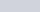. Using what we learned above, we can set up an equation.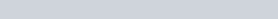There is only one variable, so we can easily solve for x.Now that we have found the abundance of 6Li, we can useto find 7Li.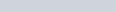So we have determined that the abundances of 6Li and 7Li are 6 % and 94 % respectively.

## Isotope Abundance Practice Problems

Problem 1

Element X has two major isotopes, one of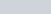at 24% abundance and another atat 76% abundance. Based solely on these two isotopes, what is the atomic weight of Element X?

Problem 2

Iron has an atomic weight of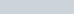with three major isotopes: 54Fe, 56Fe, and 57Fe. 56Fe has an abundance of 91.8%. What are the abundances of the other two isotopes

## Isotope Abundance Practice Problem Solutions

1:2: 7.7% 54Fe and 0.5% 57Fe